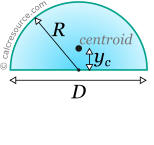## Properties of a Semi-Circular Area

This tool calculates the basic geometric properties of a semi-circular area. Enter the shape dimensions R or D below. The calculated results will have the same units as your input. Please use consistent units for any input.

 R = ...or D = Geometric properties: Radius = Diameter = Area = Perimeter = Arc length = yc =